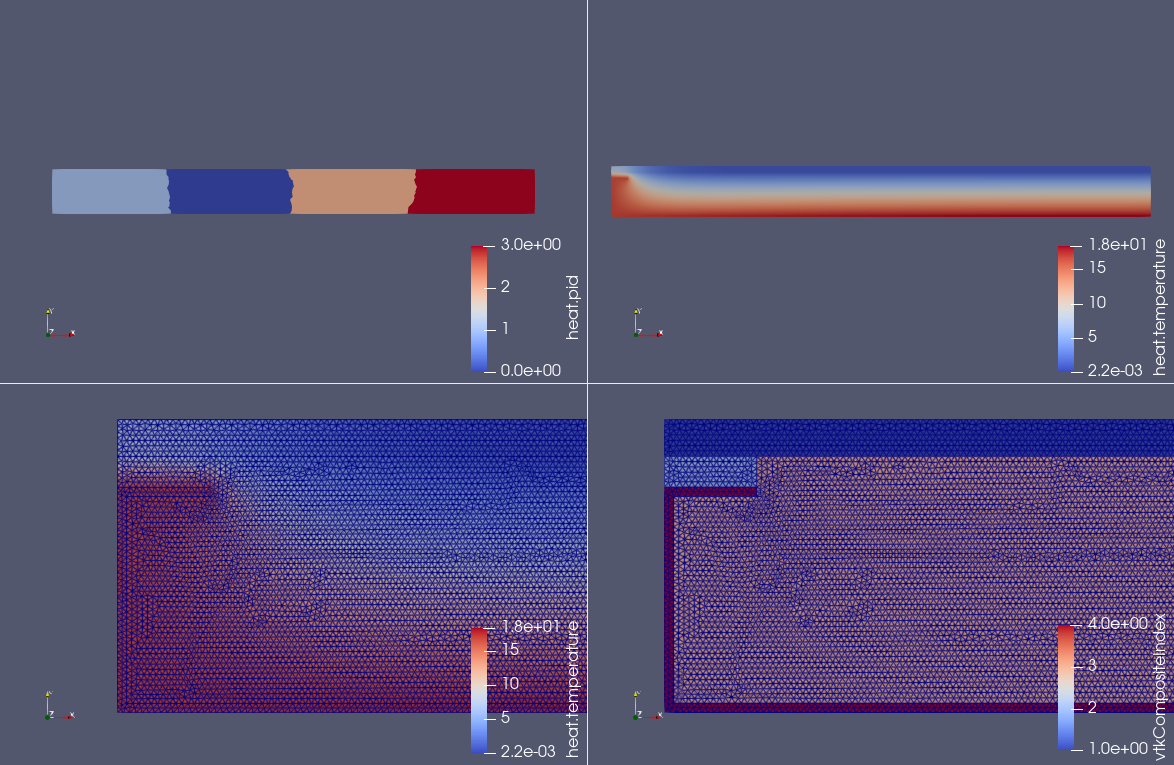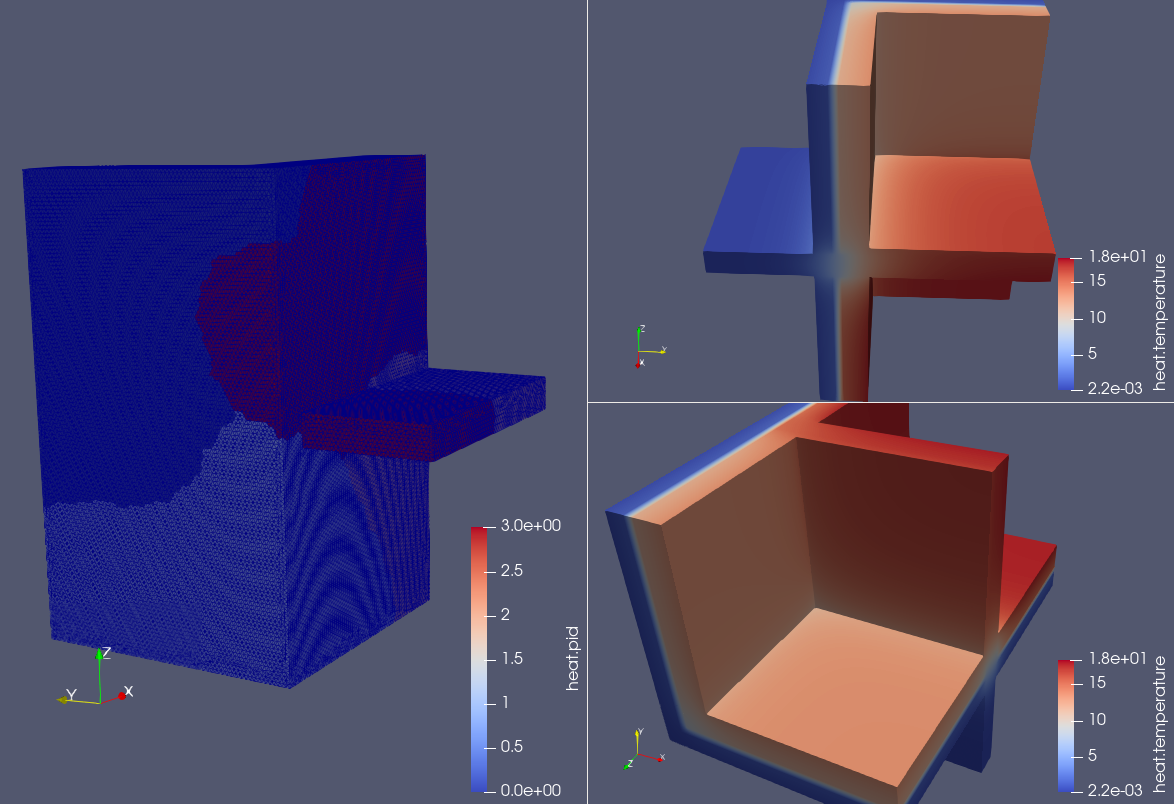# Thermal bridges in building construction

## 1. Context

ISO 10211:2007 sets out the specifications for a three-dimensional and a two-dimensional geometrical model of a thermal bridge for the numerical calculation of:

1. heat flows, in order to assess the overall heat loss from a building or part of it;

2. minimum surface temperatures, in order to assess the risk of surface condensation.

These specifications include the geometrical boundaries and subdivisions of the model, the thermal boundary conditions, and the thermal values and relationships to be used.

ISO 10211:2007 is based upon the following assumptions:

1. all physical properties are independent of temperature;

2. there are no heat sources within the building element.

ISO 10211:2007 can also be used for the derivation of linear and point thermal transmittances and of surface temperature factors. More information here.

## 2. Parameters

The parameters for this benchmark case are given by:

``````{
"Parameters": {
"h_top": "1.0/0.06",
"h_bottom": "1.0/0.11",
"T0_top": 0,
"T0_bottom": 20
}
}``````

These parameters define the top and bottom heat transfer coefficients (`h_top` and `h_bottom`) and the exterior temperatures (`T0_top` and `T0_bottom`).

## 3. Geometry and Mesh

This case uses the following mesh configuration:

2D mesh (similar in 3D)
``````{
"Meshes": {
"heat": {
"Import": {
"filename": "$cfgdir/case2.geo", "hsize": 0.001 } } } }`````` The geometry is defined in the `case2.geo` file, with a mesh size of 0.001. ## 4. Materials The materials used in the model include concrete, wood, insulation, and aluminum. The specific parameters are: ``````{ "Materials": { // material data here } }`````` Each material has specific properties including thermal conductivity (`k`), specific heat capacity (`Cp`), and density (`rho`). ## 5. Mathematical Formulation This simulation solves the steady state heat equation which can be represented by the partial differential equation (PDE): $\nabla \cdot (k \nabla T) = 0$ where: • T is the temperature, • k is the thermal conductivity. ## 6. Boundary Conditions The boundary conditions define the state of heat at the boundaries of the geometry. ``````{ "BoundaryConditions": { // boundary condition data here } }`````` The boundary conditions are given by a convective heat flux on the top and bottom of the model. The associated mathematical expressions are: For the top $-h_{\text{top}} \cdot (T - T0_{\text{top}}) = q_n$ For the bottom $-h_{\text{bottom}} \cdot (T - T0_{\text{bottom}}) = q_n$ where: • h is the heat transfer coefficient, • T is the temperature, • T0 is the exterior temperature, • q_n is the heat flux normal to the boundary. ## 7. Post-processing The post-processing step includes the extraction and analysis of data: ``````{ "PostProcess": { // post-processing data here } }`````` In the post-processing stage, several quantities are computed, including temperature fields, normal heat flux, and the heat flux at specific points. ## 8. Results Here, we present the results from the simulation.Figure 1. 2D thermal bridgeFigure 2. 3D thermal bridge: on the left the process id with the surface edges and on the right the temperature field ## 9. Running the model The configuration file is in `Building/ThermalBridgesENISO10211/case2.cfg`. The command line in feelpp-toolboxes docker or singularity reads Command line for the 2D case ``$ mpirun -np 4 feelpp_toolbox_heat --config-file case2.cfg``
Command line for the 3D case
``\$ mpirun -np 4 feelpp_toolbox_heat --config-file case3.cfg``